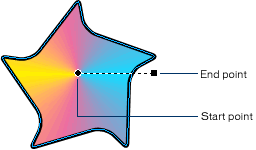Home > Products > FreeHand > Support > Learning FreeHand MX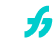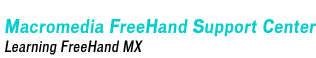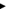Linear gradients have two handles and apply color in a straight, graduated transition in constant increments. The start point determines the location of one end of the gradient. The end point determines the location of the other end of the gradient and the gradient's angle.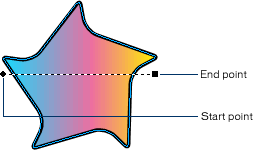Logarithmic gradients have two handles. They apply color in a straight, graduated transition using increasingly wider bands. The start point determines the location of one end of the gradient. The end point determines the location of the other end of the gradient and the gradient's angle.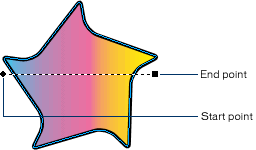Radial gradients have three handles, and apply color in concentric elliptical or circular increments, regardless of the shape of the object. The start point determines the location of the center of the gradient. The two end points determine the angle and proportions of the gradient.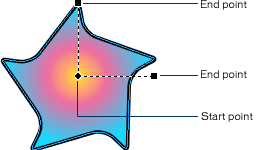Rectangle gradients are new in FreeHand MX. They have three handles and apply color in concentric rectangular or square increments, regardless of the shape of the object. The start point determines the location of the center of the gradient. The two end points determine the angle and proportions of the gradient.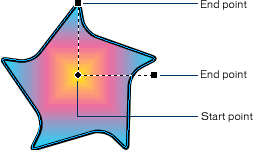Contour gradients have two handles. They apply color in concentric shapes, based on the shape of the object. The start point determines the center of the gradient. The end point determines the length of the gradient, but not its angle or shape.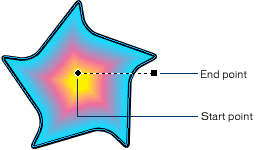Cone gradients are new in FreeHand MX. They have two handles and apply color in a 360° graduated sweep. The start point determines the center of the gradient. The end point determines the angle of the gradient, but not its length or shape.